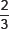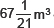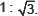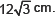# Surface Areas and Volumes-Important Questions

Class X Math
Hots for Surface Areas and Volumes
1.   An iron pillar has some part in the form of a right circular cylinder and remaining in the form of a right circular cone. The radius of the base of each of cone and cylinder is 8 cm. The cylinderical part is 240 cm high and the conical part in 36 cm high. Find the weight of the pillar if one cu. cm of iron weighs 7.8 grams.
2.   An open container made up of a metal sheet is in the form of a frustum of a cone of height 8 cm with radii of its lower and upper ends as 4 cm and 10 cm respectively. Find the cost of oil which can completely fill the container at the rate of Rs. 50 per litre. Also, find the cost of metal used, if it costs Rs. 5 per 100 cm2.
3.   A building is in the for of a cylinder surmounted by a hemispherical dome as shown in the figure. The base diameter of the dome is equal toof the total height of the building. Find the height of the building, if it containsof air.
4.   The diameter of a sphere is 28 cm. Find the cost of painting it all around at Rs. 0.10 per square cm.
5.   The perimeter of one face of a wooden cube is 20 cm. Find its weight if 1 cm3 of wood weighs 8.25 g.
6.   The radii of two cylinders are in the ratio ofIf the volumes of two cylinders be same, find the ratio of their respective heights.
7.   If the radius of the base of a cone is doubled keeping the height same. What is the ratio of the volume of the larger cone to the smaller cone?
8.   If the length, breadth and height of a solid cube are in the ratio 4 : 3 : 2 and total surface area is 832 cm2. Find its volume.
9.   Three cubes of a metal whose edges are in the ratio 3 : 4 : 5 are melted and converted into a single cube whose diagonal isFind the edges of the three cubes.
10.   A solid toy is in the form of a hemisphere surmounted by a right circular cone. The height of the cone is 4 cm and diameter of the base is 8 cm. Determine the volume of the toy. If the cube circumscribes the toy, then find the difference of the volumes of the cube and the toy. Also, find the total surface area of the toy.